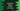# How to replace an item in an Array in JavaScript## How to replace an item in an Array in JavaScript:

In this post, we will learn how to replace an item in an array in JavaScript. We will show you different ways to do that.

### Method 1: By using the index:

This is the easiest way to replace an item in an array. Each array element can be accessed by its index. The index starts from 0, i.e. the index of the first element is 0, second element is 1 etc. If you know the index of the element you want to change, you can access it and replace it with another.

To access an element of an array, we have to use the square brackets.

Let me show you with an example:

``````let givenArray = [1, 2, 3, 4, 5];

console.log("givenArray: ", givenArray);

givenArray = -1;

console.log("After modification: ", givenArray);``````

It will print output as like below:

``````givenArray:  [ 1, 2, 3, 4, 5 ]
After modification:  [ 1, 2, -1, 4, 5 ]``````

It modifies the array in place. The third item is replaced with -1.

### Method 2: By using indexOf:

The indexOf method returns the index of an element in an array. It returns the first index of an element in an array. If the element is not found, it returns -1.

So, if we don’t know the index of an element, we can use indexOf to find the index and we can use the above method to replace that element with another.

Let me show you an example:

``````let givenArray = [1, 2, 3, 4, 5];

console.log("givenArray: ", givenArray);

let numToRemove = 4;
let index = givenArray.indexOf(numToRemove);

if (index === -1) {
} else {
givenArray[index] = -1;
}

console.log("After modification: ", givenArray);``````

Here,

• givenArray is the given array.
• numToRemove is the number to remove from the array. We are calling indexOf method to get the index of numToRemove in the array givenArray. The return value of indexOf is stored in the variable index.
• If index is -1, i.e. the number is not found in the array, it will print a message that it is not found.
• Else, it replaces the number with -1.

If you run this program, it will print the below output:

``````givenArray:  [ 1, 2, 3, 4, 5 ]
After modification:  [ 1, 2, 3, -1, 5 ]``````

If I change numToRemove to 10, it will print:

``````givenArray:  [ 1, 2, 3, 4, 5 ]
After modification:  [ 1, 2, 3, 4, 5 ]``````

### Method 3: By using splice():

The splice method can be used to remove or replace elements of an array in place. This method is defined as like below:

``splice(s, d, v1, v2, v3....vn)``

Here, s is the index to start changing the array. d is the number of elements to remove from s. v1, v2 etc. are elements to replace from index s.

If we want to replace one element in the array, we need to pass the index as s, 1 as d and the element to replace as the third argument.

Let’s try it with an example:

``````let givenArray = [1, 2, 3, 4, 5];

console.log("givenArray: ", givenArray);

givenArray.splice(2, 1, -1);

console.log("After modification: ", givenArray);``````

Here, we are passing three numbers as the arguments:

• 2 is the element index to replace.
• 1 is the number of element to replace.
• -1 is the new value.

It will print:

``````givenArray:  [ 1, 2, 3, 4, 5 ]
After modification:  [ 1, 2, -1, 4, 5 ]``````

### Method 4: Replace object properties in an array of objects:

We can use any of the above methods to replace an object with another object in an array of objects. But, if you want to replace any property of a specific object in that array, you can do that by accessing the property of that object for that index.

``````let givenArray = [
{
id: 0,
name: "Alex",
},
{
id: 1,
name: "Bob",
},
{
id: 2,
name: "Chandler",
},
{
id: 3,
name: "Daisy",
},
{
id: 4,
name: "Ela",
},
{
id: 5,
name: "Frank",
},
];

console.log("givenArray: ", givenArray);

let index = givenArray.findIndex((item) => item.name === "Ela");

if (index === -1) {
} else {
givenArray[index].name = "N/A";
}

console.log("After modification: ", givenArray);``````

In this example,

• givenArray is the given array of objects.
• It finds the index of the element with name as Ela by using findIndex.
• If it is -1, it prints a message that the item is not found.
• Else, we are using dot notation to assign N/A as the name of that object.
• The last line is printing the array content after modification.

If you run this program, it will print:

``````givenArray:  [
{ id: 0, name: 'Alex' },
{ id: 1, name: 'Bob' },
{ id: 2, name: 'Chandler' },
{ id: 3, name: 'Daisy' },
{ id: 4, name: 'Ela' },
{ id: 5, name: 'Frank' }
]
After modification:  [
{ id: 0, name: 'Alex' },
{ id: 1, name: 'Bob' },
{ id: 2, name: 'Chandler' },
{ id: 3, name: 'Daisy' },
{ id: 4, name: 'N/A' },
{ id: 5, name: 'Frank' }
]``````